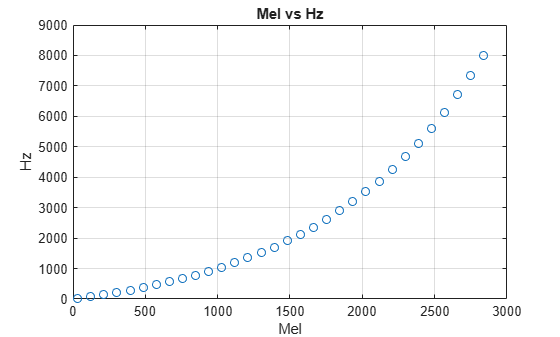# hz2mel

Convert from hertz to mel scale

## Syntax

``mel = hz2mel(hz)``

## Description

example

````mel = hz2mel(hz)` converts values in hertz to values on the mel frequency scale.```

## Examples

collapse all

Set two bounding frequencies in Hz and then convert them to the mel scale.

`b = hz2mel([20,8000]);`

Generate a row vector of 32 values uniformly spaced on the mel scale.

`melVect = linspace(b(1),b(2),32);`

Convert the row vector of values into equivalent frequencies in Hz.

`hzVect = mel2hz(melVect);`

Plot the two vectors for comparison. As mel values increase linearly, Hz values increase exponentially.

```plot(melVect,hzVect,'o') title('Mel vs Hz') xlabel('Mel') ylabel('Hz') grid on```## Input Arguments

collapse all

Input frequency in Hz, specified as a scalar, vector, matrix, or multidimensional array.

Data Types: `single` | `double`

## Output Arguments

collapse all

Output frequency on the mel scale, returned as a scalar, vector, matrix, or multidimensional array the same size as `hz`.

Data Types: `single` | `double`

## Algorithms

The frequency conversion from Hz to the mel scale uses the following formula:

`$mel=2595{\mathrm{log}}_{10}\left(1+\frac{hz}{700}\right)$`

 O'Shaghnessy, Douglas. Speech Communication: Human and Machine. Reading, MA: Addison-Wesley Publishing Company, 1987.

## Support